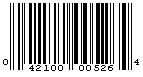# Barcodes and Modular Arithmetic

Barcodes

Here is an example of a UPC-A barcode, taken from wikipedia:UPC-A barcode exampled

A UPC-A barcode has 12 digits.  The first digit is something that tells how the numbers are generally used – for example, a particular industry might use a certain number for certain kinds of items.  The last twelfth digit is a check digit that can try to tell whether or not the numbers have an error.  This check digit is constructed in a certain way at first.  Later on, the check digit may be able to tell us if the numbers have an error or not.

The check digit is constructed as follows:

We have 11 digits:

$$ABCDEFGHIJK$$

So let $$L$$ be the last twelfth difit.  We sum the digits in the odd positions and multiply by 3, and sum that with the sum of the digits in the even positions:

$$3\cdot(A+C+E+G+I+K)+(B+D+F+H+J)$$

We take this modulo 10, or the remainder of this when divided by 10.  If this is 0, that is our twelfth digit; if not, subtract this from 10 and that is our twelfth digit.

$$\text{Let}\ S = (3\cdot(A+C+E+G+I+K)+(B+D+F+H+J))$$

\begin{equation}
L=
\begin{cases}
0, & \text{if}\ S \pmod{10} \equiv 0 \\
10 – (S \pmod{10}), & \text{otherwise}
\end{cases}
\end{equation}

So the logic is that if all 12 digits are correct, they satisfy the check digit equation:

$$3\cdot(A+C+E+G+I+K)+(B+D+F+H+J+L) \equiv 0 \pmod{10}$$

If there is an error in the 12th digit, of course the check digit equation won’t be satisfied.  If there is an error in any one single digit among the first 11 digits, then the check digit equation will also not be satisfied.  Thus, the check digit equation will detect any single digit error.

To see that a single digit error among the first 11 digits will cause the check digit equation to not be satisfied, first note that if any of the digits in the even position are off, that will manifest in $$S$$ as well as $$S \pmod{10}$$ and we will have $$S \pmod{10} \not\equiv 0$$.  But what about the digits in the odd positions, whose sum is multiplied by 3, and why multiplied by 3?

Take a digit in one of the even positions.  As long as the digit is off from the correct value, that will manifest itself in $$S$$ and $$S \pmod{10}$$.  Now take a digit in one of the odd positions and call id $$O$$.  The question then is, if the digit is off from the correct value by say $$d$$, how will that manifest itself in $$S$$ as well as $$S \pmod{10}$$?  The correct $$O$$ gives a term $$3 \cdot O$$ in $$S$$ while an incorrect digit of say $$O + d$$ gives a term $$3 \cdot O + 3 \cdot d$$.